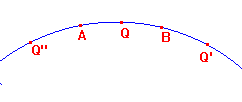proof of lemma

 Lemma Suppose that A, B and Q lie on a hyperbolic line H with d(A,B) = t > 0. Then (1) e-t < cosh(d(A,Q))/cosh(d(B,Q) < et, and (2) for s ε (e-t,et), there is a unique Q on H with cosh(d(A,Q))/cosh(d(B,Q)) = s. Proof of Lemma Let a = d(A,Q), b = d(B,Q). Observe that, for x ≥ 0, 0 ≤ tanh(x) < 1.(1) There are really three case, (a) Q beyond B (e.g. Q' in figure). Here a = b+t, so, by the Addition Formula for cosh, cosh(a)/cosh(b) = cosh(t)+tanh(b)sinh(t) Using the inequalities for tanh(b), cosh(t) ≤ cosh(a)/cosh(b) < cosh(t)+sinh(t) = et. (b) Q between A and B (e.g. Q in figure). Here a = t-b, with b ≤ a, so we get cosh(a)/cosh(b) = cosh(t)-tanh(b)sinh(t). Using the inequalities 0 ≤ tanh(b) ≤ tanh(t), cosh(t)-tanh(t)sinh(t) < cosh(a)/cosh(b) ≤ cosh(t). Note that, from the hypertrigonometric identities, cosh(t)-tanh(t)sinh(t)=1/cosh(t). Thus, in this case 1/cosh(t) ≤ cosh(a)/cosh(b) ≤ cosh(t). (c) Q beyond A (e.g. Q" in figure). If we reverse the roles of A and B and use the argument of (a), cosh(t) ≤ cosh(b)/cosh(a)